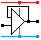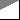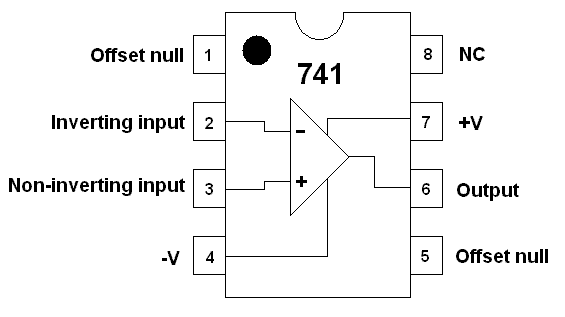Home

rOm
Quest
Glossary

Random
Page
Search
Site
Lush
Sim
Class
Subject
Images

Help
FAQ
Sign
Up
Log
In
 AS Level     >Op Amp<     Delta Lux     Gain     Schmitt Astable     Schmitt Trigger     Virtual Earth     Voltage Follower

# Op Amp

Site for Eduqas/WJEC - Go to the AQA site.

AS Level    Op AmpsQuestions 0 to 5   -->  View All

Operational amplifiers have a very high open loop gain, a high input impedance and a low output impedance.

Amplifiers increase the magnitude of the input signal.

With this amplifier, the output voltage is bigger and it's inverted.## Amplifiers have one or more of the following properties.

• The output voltage may be bigger than the input voltage
• The output current may be bigger than the input current
• The output power may be bigger than the input power
• High input impedance (AC equivalent of resistance)
• Low output impedance (AC equivalent of resistance)

## The 741 Operational AmplifierPins

1. Offset Null - Rarely used. It can be used to to adjust for small errors in the two inputs so zero volts in gives zero volts out.
2. Inverting Input - If this voltage goes up, the output voltage will go DOWN unless the Op Amp is already saturated.
3. Non-Inverting Input - If this voltage goes up, the output voltage will go UP unless the Op Amp is already saturated.
4. The minus supply - Sometimes this is connected to zero volts ( ground ).
Sometimes it's connected to a voltage between -5V and -18V or more for a few specialised op amps.
5. Offset Null - Rarely used. It can be used to to adjust for small errors in the two inputs so zero volts in gives zero volts out.
6. The Output - In an ideal Op Amp, the maximum and minimum output voltage is equal to the power supply voltages.
In a real life Op Amp, these voltages are 2 to 3 Volts less.
7. The plus supply - Voltages from +5V to +18V are common.
There are specialist and more expensive Op Amps with a higher voltage ratings.
8. No Connection - This pin is not used.

The 741 is a low cost operational amplifier. It has ...

• A very high open loop gain (close to infinity)
• Very high input resistance (close to infinity)
• Low output resistance (close to zero)
• A rather poor gain bandwidth product compared with slightly more expensive op' amp's.
• It's very easy to use. Higher performance devices often suffer from unwanted parasitic oscillation and are much harder to work with. A technique called equalisation prevents this.

It can be used to make ...

• EVERYONE
• Comparators (compare two voltages)
• Inverting Amplifiers (make the input signal bigger and invert it)
• Non Inverting Amplifiers (make the input signal bigger without inverting it)
• Summing Amplifiers (add two or more input voltages and amplify and invert the result)
• AS Level
• Voltage Follower. A non-inverting amplifier with a high power or current gain and a voltage gain of one.
• Schmitt Trigger (compare the input with two different reference voltages).
• A Level
• Difference (subtracting) Amplifiers (subtract two input voltages and amplify the result)

## Feedback Resistors >= 1k and <= 1M

• These are usually in the range 1k to 1M.
• Resistors less than 1k might lead to currents greater than a typical op-amp can supply.
• Resistors greater than 1M might allow op-apm leakage currents to become important and the circuit will not beave as expected.
• There are specialised op-apms for instrumentation or power systems where these limits don't apply.

## Operational Amplifier Ideal and Typical Properties

 Characteristics Ideal Real - Depends on the model GAIN: Open Loop Infinity > 100000 or 1000000 typical Input Resistance: (Impedance) Infinity > 1M (Bipolar) > 1000M (FET) Output Resistance: (Impedance) Zero 100 - 1000R SATURATION High: Highest Output Voltage Positive Power Supply Voltage Typical value = 12 V. Two volts below the positive supply Typical value = 10 V. SATURATION Low: Lowest Output Voltage Negative Power Supply Voltage Typical value = -12 V. Two volts above the negative supply Typical value = -10 V. Gain Bandwidth Product: Infinity 1 - 20MHz Input offset voltage: Zero Often Adjustable to Zero

Subject Name     Level     Topic Name     Question Heading     First Name Last Name Class ID     User ID

 CloseEscapeX Q: qNum of last_q     Q ID: Question ID         Score: num correct/num attempts         Date Done

Question Text

image url

Help Text
Debug

• You can attempt a question as many times as you like.
• If you are logged in, your first attempt, each day, is logged.
• To improve your scores, come back on future days, log in and re-do the questions that caused you problems.
• In the grade book, you can delete your answers for a topic before re-doing the questions. Avoid deleting unless you intend re-doing the questions very soon.